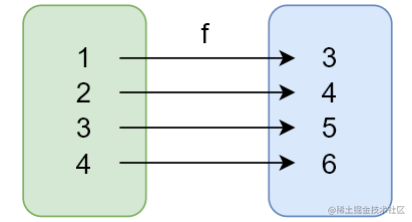什么是函数式编程？

1. 什么是函数式编程
2. 为什么要学习函数式编程
3. 函数式编程的特性
4. 函数式编程应该怎么用，用在哪里
5. 怎样提高函数式编程的效率（避免重复造轮子）

什么是函数式编程？

• 面向对象编程： 把现实世界的事物和事务抽象成程序世界中的类和对象，通过封装继承多态来演示事物事件的联系。
• 函数式编程： 把现实世界的事物和事物之间的联系抽象到程序世界，抽象的是运算过程。函数式编程中的函数不是程序中的函数，而是数学中的函数（映射关系），例如：y = sin(x). 函数式编程用来描述数据之间的映射，相同的输入始终要得到相同的输出。

// 面向过程
let num1 = 1;
let num2 = 2;
let sum = num1 + num2;
console.log(sum)

//函数式
return n1 + n2;
}
console.log(sum)

为什么要学习函数式编程

• React、Vue 开始拥抱函数式编程，函数式编程的技能会是工作中必备的技能
• 抛弃 this，相同输入一定得到相同输出，专注计算和过程，让代码更容易理解
• 方便测试、方便问题定位、方便并行处理
• 代码优化：打包过程中，可以更好的利用 tree shaking 过滤无用代码
• 有丰富的轮子：lodash、underscore、ramda

函数式编程的特性

• 函数是一等公民
• 高阶函数
• 闭包

函数是一等公民

MDNFirst-class Function（头等函数） - 术语表 | MDN (mozilla.org)

• 函数可以存储在变量中
• 函数作为参数
• 函数作为返回值

• 把函数赋值给变量
// 把函数赋值给变量
let fn = function () {
console.log('Hello First-class Function')
}
fn()

// 一个示例
const BlogController = {
index (posts) { return Views.index(posts) },
show (post) { return Views.show(post) },
create (attrs) { return Db.create(attrs) },
update (post, attrs) { return Db.update(post, attrs) },
destroy (post) { return Db.destroy(post) }
}

// 优化后，将 Views 的方法直接赋值给方法
const BlogController = {
index: Views.index,
show: Views.show,
create: Db.create,
update: Db.update,
destroy: Db.destroy
}

高阶函数

什么是高阶函数

• 函数作为参数
// forEach
function forEach(array,fn){
for(let i = 0; i < array.length; i++){
fn(array[i])
}
}

// filter
function filter(array, fn){
let result = [];
for (let i = 0; i < array.length; i++){
if(fn(array[i])){
result.push(array[i])
}
}
return result
}

//forEach
let arr1 = [1,2,3,4,5,6];

forEach(arr1, function(item){
console.log(item)；
})

//filter
let arr2 = [1,2,3,4,5,6]
let r = filter(arr2, function(item){
return item % 2 == 0;
})
console.log(r)

• 函数作为返回值
function makeFn(){
let msg = 'hi funciton';
return function(){
console.log(msg)
}
}

const fn = makeFn();
fn();
makeFn()();

//once
function once(fn){
let done = false;
return function(){
if(!done){
done = true;
return fn.apply(this, arguments)
}
}
}
let pay = once(function (money){
console.log(`支付：\${money}元`)
})

//只会执行一次
pay(10);
pay(10);
pay(10);

使用高阶函数的意义

1. 抽象可以帮助我们屏蔽细节，只需要关注我们想要实现的目标
2. 高阶函数可以用来抽象通用的问题，减少重复代码

常用的高阶函数

forEach，map，filter，every，some，find/findIndex，redouce，sort

const map = (array, fn) => {
let result = [];
for (const item of array) {
result.push(fn(item))
}
return result
}

const every = (array, fn) => {
let result = true;
for (const item of array) {
if (!fn(item)) {
result = false;
break
}
}
return result
}

const find = (array, fn) => {
let result;
for (const item of array) {
if (fn(item)) {
result = item
break
}
}
return result
}
const reduce = (array, fn, initialValue) => {
if (!array.length) {
return;
}
let res = initialValue || array;
for (let i = initialValue ? 0 : 1; i < array.length; i++) {
res = fn(res, array[i], i, array)
}
return res
}

let a = [1, 2, 4, 3, 4, 2, 5, 6]
console.log(every(a, item => item > 0))
console.log(map(a, item => item + 1))
console.log(find(a, item => item > 3))
console.log(reduce(a, (item1, item2) => item1 + item2,10))
console.log(reduce([1,2,3,4,4,5,5,7,1,5,4,8],(prev,item)=>{
!prev.includes(item) && prev.push(item);
return prev;
},[]))

闭包

• 可以在另一个作用域中，调用一个函数的内部函数，并访问到该函数作用域中的成员。
// 函数作为返回值
function makeFn () {
let msg = 'Hello function'
return function () {
console.log(msg)
}
}
const fn = makeFn()
fn()

//fn 可以访问到 makeFn 中的匿名函数，并且可以访问到 makeFn 作用域中的 msg 变量

• 闭包的本质：函数在执行的时候会放到一个执行栈上当函数执行完毕之后会从执行栈上移除，但是 堆上的作用域成员因为被外部引用不能释放，因此内部函数依然可以访问外部函数的成员
// 生成计算数字的多少次幂的函数
function makePower (power) {
return function (x) {
return Math.pow(x, power)
}
}
let power2 = makePower(2)
let power3 = makePower(3)
//类似于工厂模式，返回多种具有特定功能的函数

纯函数• slice 返回数组中的指定部分，会返回一个新数组，不会改变原数组

• splice 对数组进行操作，并返回该数组，会改变原数组

函数式编程的好处

1. 可缓存： 纯函数对相同的输入始终有相同的输出，所以把纯函数的结果缓存起来

function memoize(f) {
let cache = {}
return function () {
let key = JSON.stringify(arguments);
cache[key] = cache[key] || f.apply(f, arguments);
return cache[key]
}
}

function getArea(r) {
console.log(r);
return Math.PI * r * r
}

let f = memoize(getArea);

console.log(f(6))
console.log(f(6))

// 通过 memoize 函数，将 getArea 函数相同 arguments 的函数处理返回值以 arguments 字符串为 key，缓存到 cache 对象中，下次再次调用相同argument 参数的 getArea function 时，直接读取缓存，不再重新计算
复制代码
2. 可测试

纯函数相同输入相同输入，可以更加方便的测试

3. 并行处理

纯函数不需要访问共享的内存数据，在并行的环境下（Web Worker），可以任意运行纯函数

副作用

// 不纯的
let mini = 18
function checkAge (age) {
return age >= mini
}

// 纯的(有硬编码，后续可以通过柯里化解决)
function checkAge (age) {
let mini = 18
return age >= mini
}

• 配置文件
• 数据库
• 用户输入